GFG App
Open AppBrowser
Continue

# Mode: Meaning, Formula, Merits, Demerits, and Examples

The word mode is derived from the French word ‘La Mode’, meaning anything that is in fashion or vogue. A measure of central tendency in statistical series that determines the value occurring most frequently in a given series is known as mode. In other words, the modal value of the series has the highest frequency in the given series. For example, if in a class of 100 students, 20 students have opted for Mathematics, 50 students have opted for IP, and the rest 30 have opted for Physical Education, then the modal opted subject of the class would be IP.

Mode is represented by the letter Z.

According to Kenny, “The value of the variable which occurs most frequently in a distribution is called the mode.”

According to Croxton and Cowden, “The mode may be regarded as the most typical of a series of value.”

1. Mode is extensively used by investigators for measuring the taste and preferences of people for a specific brand of commodity.

2. If there is frequency distribution series, then to determine the mode, the value corresponding to the maximum frequency is taken.

3. In a case where the concentration of observations around a value with maximum frequency is less than the concentration of observations around any other value, then it is not possible to calculate the mode by using the maximum frequency criterion. For example, in the below frequency distribution table, frequency of 26 is the maximum corresponding to 55 marks. Hence, according to the maximum frequency criterion, the modal marks of the series should be 55. However, the highest concentration of the frequency is around 70 marks. The frequencies around 70 are more; i.e., 18, 20, and 22 as compared to neighbourhood frequencies of 55; i.e., 2, 26, and 6. Hence, the correct definition of mode can be the value of observation around which other items of the given distribution are denser.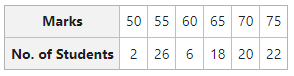4. When the investigator wants to know the most typical value of a given set, then the mode is a preferable measure of central tendency than mean and median. For example, the most common garment size, the most popular celebrity in Bollywood, the more common pocket money of a student, etc.

5. A given distribution can either be uni-modal, bi-modal, or multi-modal. Also, there can be a situation of no mode when each item of the series occurs an equal number of times.

• No Modal Value: When each observation of a series occurs the same number of times. For example, for the series 3, 4, 5, 4, 3, 5, 2, 2 there is no modal value as every item is occurring 2 times.
• Uni-modal: When one observation or item of a series occurs the maximum number of times. For example, the modal value or mode of the series 2, 4, 2, 5, 2, 6, 8 is 2 as it is occurring for the maximum number of times.
• Bi-modal: When two observations or items of a series have the same maximum frequency. For example, the mode of the series 4, 5, 4, 6, 5, 7, 8, 9 is 4 and 5 as both of these items are occurring two times.
• Multi-modal: When more than two observations or items of a series have the same maximum frequency. For example, the mode of the series 2, 3, 4, 4, 5, 5, 2, 1, 1, 7, 6, 9, 8 are 2, 4, 5, and 1.

Mode with Frequency Curve

If the investigator wants to explain the mode of a series graphically, then the mode of that series would be the point of maximum frequency and is indicated by the peak of a frequency curve. In the graph given below, the X-axis denotes the value of variable and the Y-axis denotes the corresponding frequencies. Now, mode will be that value on the X-axis, with the corresponding maximum frequency on the Y-axis.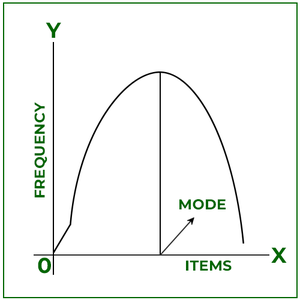### Calculation of Mode

Mode is calculated in three different types of series; viz., Individual, Discrete and Continuous Series.

### Individual Series

The mode of an individual series is the value that occurs the most frequently and can be determined by two methods, the Inspection Method and by converting Individual Series into Discrete Frequency Series.

#### Example:

The age of a class of 12 students is given below. Find the modal age of the class.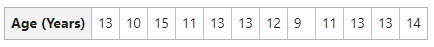#### Solution:

By Inspection Method,

The age 13 occurs most frequently in the given series (5 times).

Therefore, Mode (Z) = 13

### Discrete Series

In a discrete series, values are given with their respective frequencies. The mode of discrete series is the value whose frequency has occurred most frequently in a given series. It can be determined by two methods, the Inspection Method and Grouping Method.

#### Example:

The table below shows the number of sixes in the cricket taken by a batsman in 12 matches. Determine the mode of the provided data set.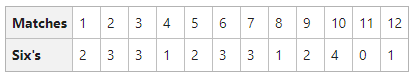#### Solution:

By Inspection Method:

Here 3 is repeated the most.

Therefore, Mode (Z) = 3

### Continuous Series

In the case of a continuous frequency distribution, the modal class is the one with the highest frequency. It can be determined by two methods, the Inspection Method and Grouping Method. The formula for calculating mode by both methods isHere,

Z = Value of Mode= lower limit of the modal class= frequency of modal class= frequency of pre-modal class= frequency of the next higher class or post-modal class

i = size of the modal group

Modal class is the class interval with the highest frequency in the series.

#### Example:

Calculate the value of mode for marks obtained by the students from the table below.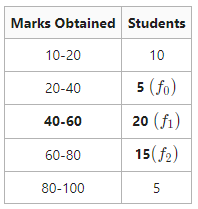#### Solution:

By Inspection method:

The Class Interval 40-60 has the highest frequency, i.e., 20. Therefore, the modal class of the given series is 40-60, which means that 20 is the frequency of the modal class, 5 is the frequency of the pre-modal class, and 15 is the frequency of the post-modal class.= 55

Therefore, Mode (Z) = 55

### Merits of Mode

Some of the advantages of mode are as follows:

1. Simple and Popular: Mode is a simple measure of central tendency, as one can easily determine the modal value by just glancing at the given series. Its simplicity makes it a very popular measure of central tendency.

2. Graphic Location: One can even locate the mode of a given series through the graph. it can be done with the help of a histogram.

3. No need to know all the Items or Frequencies: To calculate or determine the mode of a given series, one does not require to have knowledge of all the items or frequencies of a series. In simple series, the value with the highest frequency is enough for determining the mode.

4. Least effect of Marginal Values: As compared to the mean, the mode is the measure of central tendency that carries with it the least effect of the marginal values of a series. It is because the mode of a series is determined only through the highest frequencies.

5. Best Representative Value: Mode is that value of a series that occurs most frequently. Therefore, it shows the best representative value of the given series.

6. Possible in the case of Open-end Series: One can determine the mode in open-end distributions without ascertaining the class limits.

7. Useful for both Quantitative and Qualitative Data: One can determine the mode to describe the quantitative as well as qualitative data.

### Demerits of Mode

Some of the demerits of the mode are as follows:

1. Uncertain and Vague: Amongst the other measures of central tendency, the mode is vague and uncertain.

2. Difficult: When the frequency of all items in a statistical series is identical, it is difficult for the investigator to identify the modal value of that series.

3. Not capable of further Algebraic Treatment: Unlike mean, one cannot perform further algebraic treatment with mode.

4. Complex Procedure of Grouping: Calculating the mode of a statistical series sometimes also includes the grouping of data, which can be cumbersome, making it difficult to determine the modal value. Also, if the extent of the group changes, it will also change the modal value of the series.

5. Ignores Extreme Marginal Frequencies: The calculation of mode does not consider extreme marginal frequencies. It means that mode is not a representative value of all the values in a given series.

My Personal Notes arrow_drop_up